# The electrical resistance as a component

## Why a color code for electrical resistors?

While the resistance value can be printed on large, high-power resistors, small resistors do not provide space for writing. Therefore, marking with colored rings was introduced for them.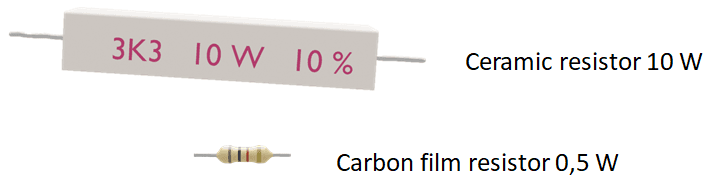Electrical resistors of different sizes

### Meaning of the colors in the resistance code

The color rings are used to indicate the following values:

Resistance value in ohms– 2 or 3 color rings, depending on the E-series.

Multiplier: Equivalent to multiplying by a power of ten. This multiplier will basically shift your decimal place around to change your value from mega ohms to milliohms and anywhere in between.

Tolerance: How much can the actual resistance value deviate from the specified value.

Resistors are usually available with 4 or 5 color rings. In general, the lower the tolerance of the resistance value, the more accurate the resistance value can be specified, i.e. one digit more is required. Example: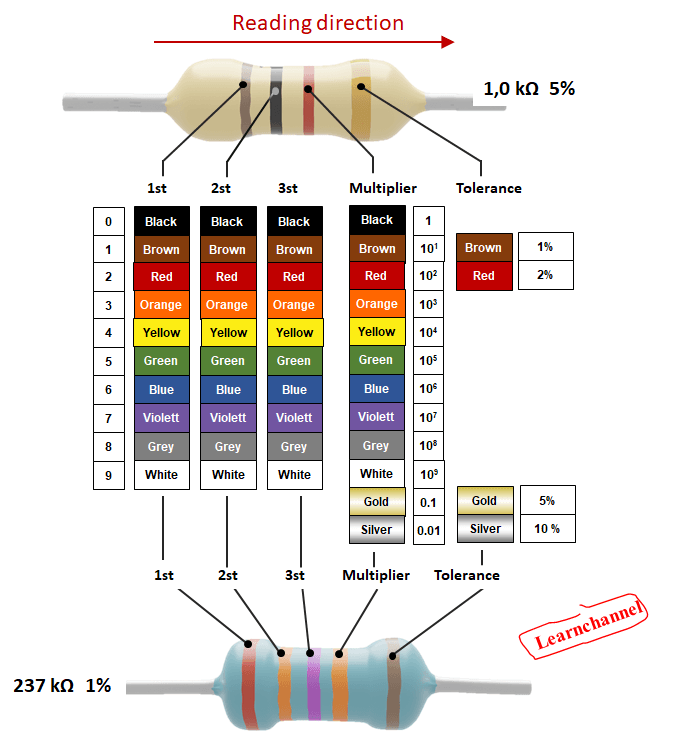Resistor color code - how it works

## Meaning of the E-resistance series:

The E resistor series were developed to make the number of resistor values manageable.

The number and gradation of the individual resistor values depend on the tolerance of the resistor. That is, the lower the tolerance of the resistors, the higher the number of gradations within a decade.

There are the E-series E3, E6, E12, E24, E48, E96 and E192. Using the E-series E12, E24 and E48 as an example, we want to show the principle of how this gradation of resistance: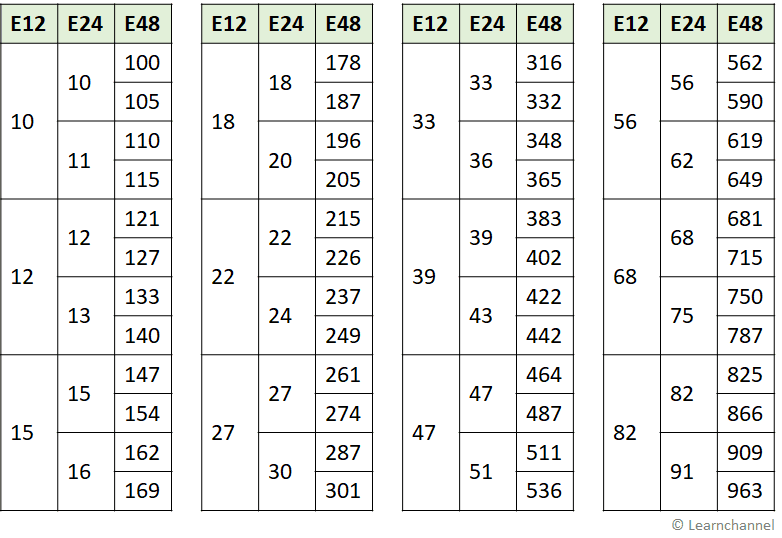Summary of EIA preferred or Standard Resistor Value Series

The resistors of the E12 and E48 series have 4 rings (2 rings for the valence, multiplier and tolerance 1 ring each).

In the E48 series there are 5 rings, because 3 rings are used for the number of the valence. You can already see from the table that the resistance values from the E48 series are given as a 3-digit number.

Note: EIA stands for Electrical Industries Association

### How the resistors get their values:

Each resistor value must be so large that it is larger than the preceding resistor by a certain factor r. This factor r is constant through the whole E-series from resistance value to the next higher resistance value.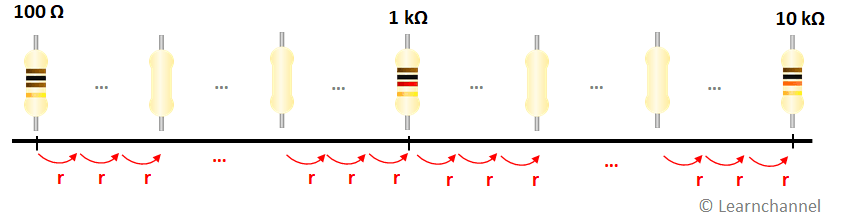Constant distance of the resistance values of the E-series

The factor r is calculated from the Xth root of 10. X is the number of resistors of a decade. So, for the E3 series X = 3, for the E12 series X = 12, etc....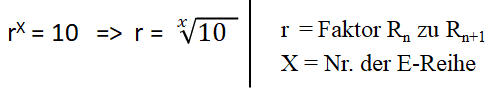Formula for r of an E series

This results in the resistance values for the resistors between the powers of ten.

For the E12 series, r = 1.21. For example, how the first two resistance values are related to each other: 1.0 * 1.21 = 1.2 and 1.2 x 1.21 = 1.5 (rounded in each case):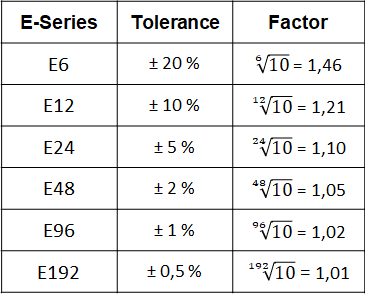E-series Resistance values - Tolerance and r-value